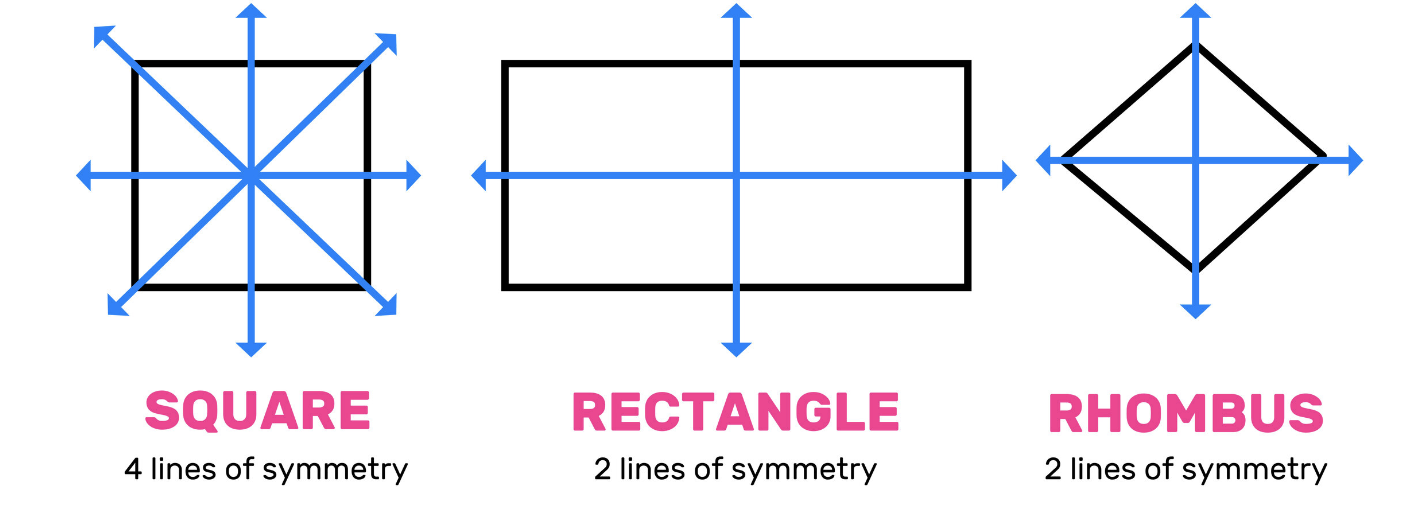# What is a Line of Symmetry?In mathematics, lines of symmetry are imaginary lines that are drawn through a symmetrical shape. This line divides each part of the shape into two halves that match exactly. This line is also known as the mirror line or the axis of symmetry. A line of symmetry depends on the geometrical shape of the object being examined. For example, the Star of David has 6 lines of symmetry, while a rectangle has 2 such lines.

## Categories of the line of symmetry

You can easily categorize the different lines of symmetry based on their orientation. This is because different geometrical shapes have various symmetrical axes through which this imaginary asymmetrical line passes. The line of symmetry can be classified into three main types, such as:

• Horizontal lines of symmetry: Horizontal lines of symmetry are imaginary horizontal lines that divide an object into two halves. Horizontal lines of symmetry run from the right to the left side $or vice versa$ of the object.
• Vertical lines of symmetry: Vertical lines of symmetry are imaginary vertical lines that divide a shape into two halves. These vertical lines usually run from the bottom to the top $or vice versa$ of the object.
• Diagonal lines of symmetry: Diagonal lines of symmetry are imaginary diagonal lines that divide a shape into two identical halves. This is a slanting imaginary line that can be used to divide the shape into two equal parts.

## Lines of symmetry and geometric shapes

Lines of symmetry are used best when describing the symmetrical or asymmetrical nature of geographic shapes. Since different geometric shapes have different symmetrical properties, it means that they have different lines of symmetry. These are the total number of lines of symmetry that we have for various shapes: Scalene or irregular triangles: This is a triangle that does not come with two identical sides. It does not have any lines of symmetry.

• Equilateral triangle: As a triangle that has all of its sides equal, you can find three lines of symmetry with this shape. These lines all intersect at the midpoint of the triangle.
• A square: A square has four identical sides. This means that the shape has 4 lines of symmetry. Each of these imaginary lines touches the midpoint. Pentagon: A Pentagon has five equal sides. This same shape is known to have five lines of symmetry. Each of these lines can be drawn through the vertex of the shape and the midpoint on the opposite side.
• A circle: A circle has an unlimited number of lines of symmetry.
• Semi-circle: A semi-circle comes with a single line of symmetry.
• Parallelogram: A parallelogram is an asymmetrical shape that does not have any lines of symmetry.### How to practice lines of symmetry

If you are looking for the answer to the question, “What is a symmetry line?” then you may want to rely on the MathMaster app. MathMaster is a math-solving tool that has the answers to basic questions like “What is symmetry line?” You can also find a wide range of answers about the subject on this tool. With this tool, you can easily practice a wide range of basic mathematical problems. Lines of symmetry are a simple mathematical problem that can be solved the right way with the right tools.

### FAQs

What is symmetry line? An asymmetry line is an imaginary line that can be used to divide a symmetrical shape into two equal parts. Many symmetrical shapes have various lines of symmetry. An asymmetrical shape does not have any lines of symmetry.
What is a symmetrical shape? A symmetrical shape is a geometrical object that can be divided into equal parts by drawing lines of symmetry through them. Many symmetrical shapes, like triangles, squares, pentagons, and hexagons, have multiple lines of symmetry.
What are asymmetrical shapes? These are geometrical shapes that do not have any lines of symmetry. In practice, these objects are said to have zero symmetry lines. Asymmetrical shapes have zero lines of symmetry because they have no symmetrical axes.